# Factoring Review Factoring Chart This chart will help

• Slides: 13Factoring Review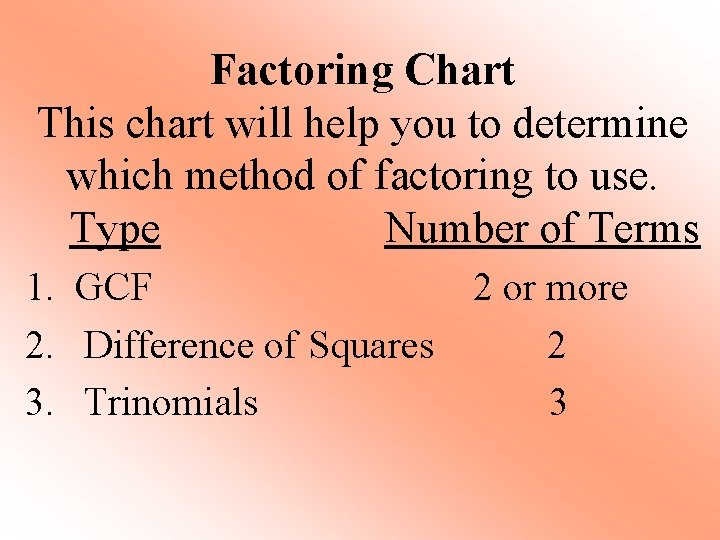Factoring Chart This chart will help you to determine which method of factoring to use. Type Number of Terms 1. GCF 2 or more 2. Difference of Squares 2 3. Trinomials 3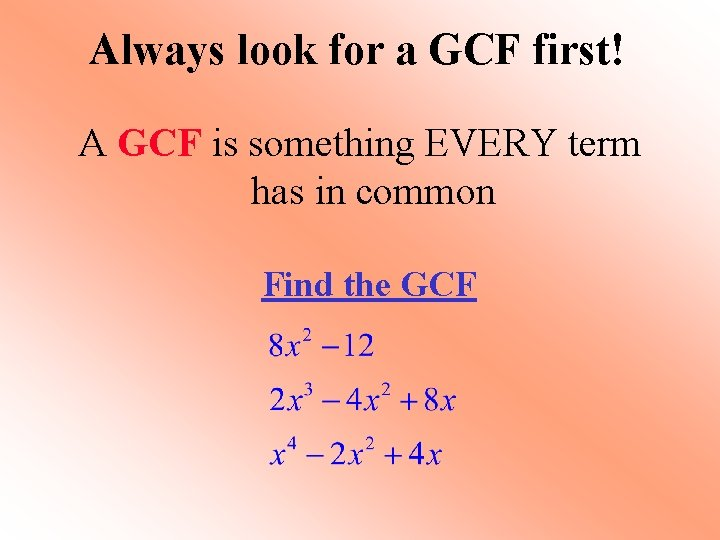Always look for a GCF first! A GCF is something EVERY term has in common Find the GCF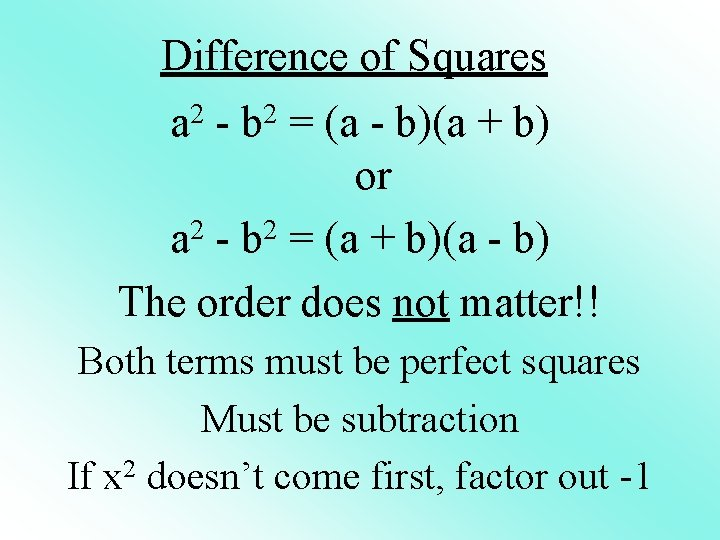Difference of Squares a 2 - b 2 = (a - b)(a + b) or 2 2 a - b = (a + b)(a - b) The order does not matter!! Both terms must be perfect squares Must be subtraction If x 2 doesn’t come first, factor out -1Factor x 2 - 25 Do you have a GCF? No Are the Difference of Squares steps true? Two terms? Yes 1 st term a perfect square? Yes 2 nd term a perfect square? Yes Subtraction? Yes x 2 – 25 ( x + 5 )(x - 5 )Factor 16 x 2 - 9 Do you have a GCF? No Are the Difference of Squares steps true? 16 x 2 – 9 Two terms? Yes 1 st term a perfect square? Yes 2 nd term a perfect square? Yes Subtraction? Yes (4 x + 3 )(4 x - 3 ) x= 4/3, -4/3Factor 3 36 x-49 x Do you have a GCF? Yes! GCF = x x(36 -49 x 2) -x(49 x 2 -36) Are the Difference of Squares steps true? Two terms? Yes 1 st term a perfect square? Yes 2 nd term a perfect square? Yes Subtraction? Yes -x(49 x 2 – 36) -x(7 x+ 6 )(7 x - 6 )Factor 50 x + 2 7 x You cannot factor using difference of squares because there is no subtraction! But you can still look for GCF x(50+7 x)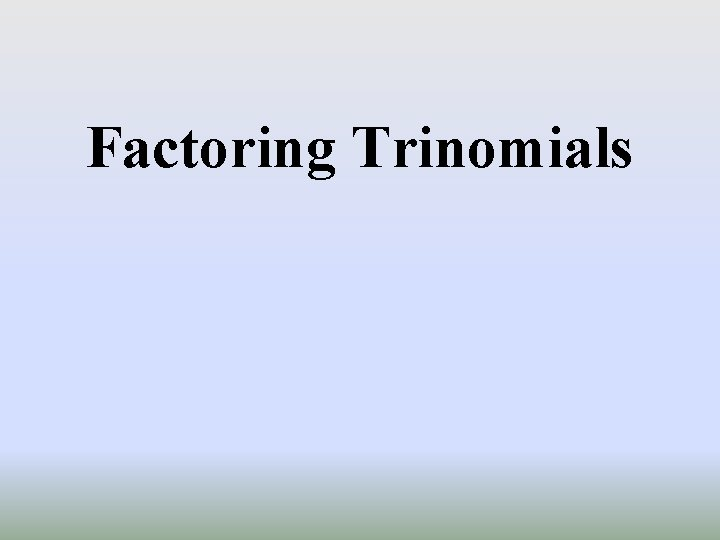Factoring TrinomialsFactoring Trinomials Step 1: Make sure everything is on one side of the equation Step 2: Multiply 1 st term by last term Step 3: Set up ( ) for factors and divide by 1 st term Step 4: Find 2 numbers that multiply to last term and add to middle term Step 5: Simplify fractions, if they do not simplify, bring denominator to the front Step 6: Set equal to 0 and solve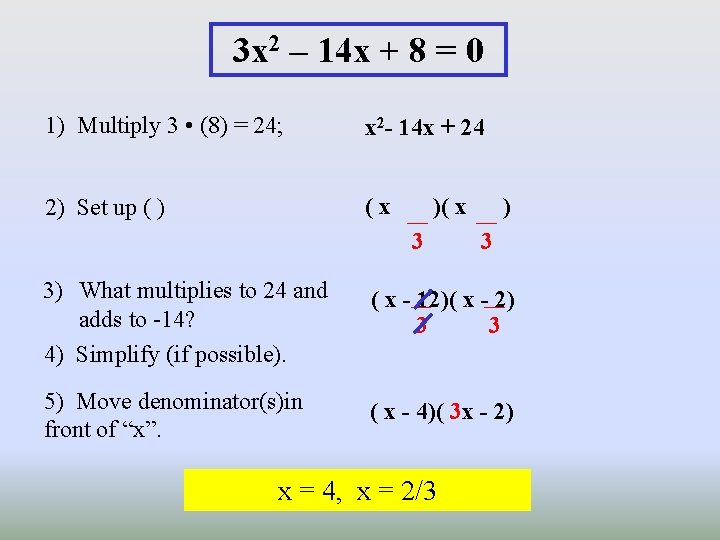3 x 2 – 14 x + 8 = 0 1) Multiply 3 • (8) = 24; x 2 - 14 x + 24 2) Set up ( ) (x )( x 3 ) 3 3) What multiplies to 24 and adds to -14? 4) Simplify (if possible). ( x - 12)( x - 2) 3 3 5) Move denominator(s)in front of “x”. ( x - 4)( 3 x - 2) x = 4, x = 2/32 x 2 – 3 x – 9 = 0 1) Multiply 2 • (-9) = -18; x 2 - 3 x - 18 2) Set up ( ) (x )( x 2 ) 2 3) What multiplies to -18 and adds to -3? 4) Simplify (if possible). ( x - 6)( x + 3) 2 2 5) Move denominator(s)in front of “x”. ( x - 3)( 2 x + 3) x = 3, x = -3/26 x 3 + 13 x 2 = -6 x 1) Rewrite and factor GCF x(6 x 2 + 13 x + 6) = 0 2) Multiply 6 • (6) = 36; x(x 2 + 13 x + 36) 3) Set up ( ) x( x )( x 6 ) 6 4) What multiplies to 36 and adds x( x + 4)( x + 9) to 13? 6 6 5) Simplify (if possible). 5) Move denominator(s)in front of “x”. x(3 x + 2)( 2 x + 3) x=0, x = -2/3, x = -3/2